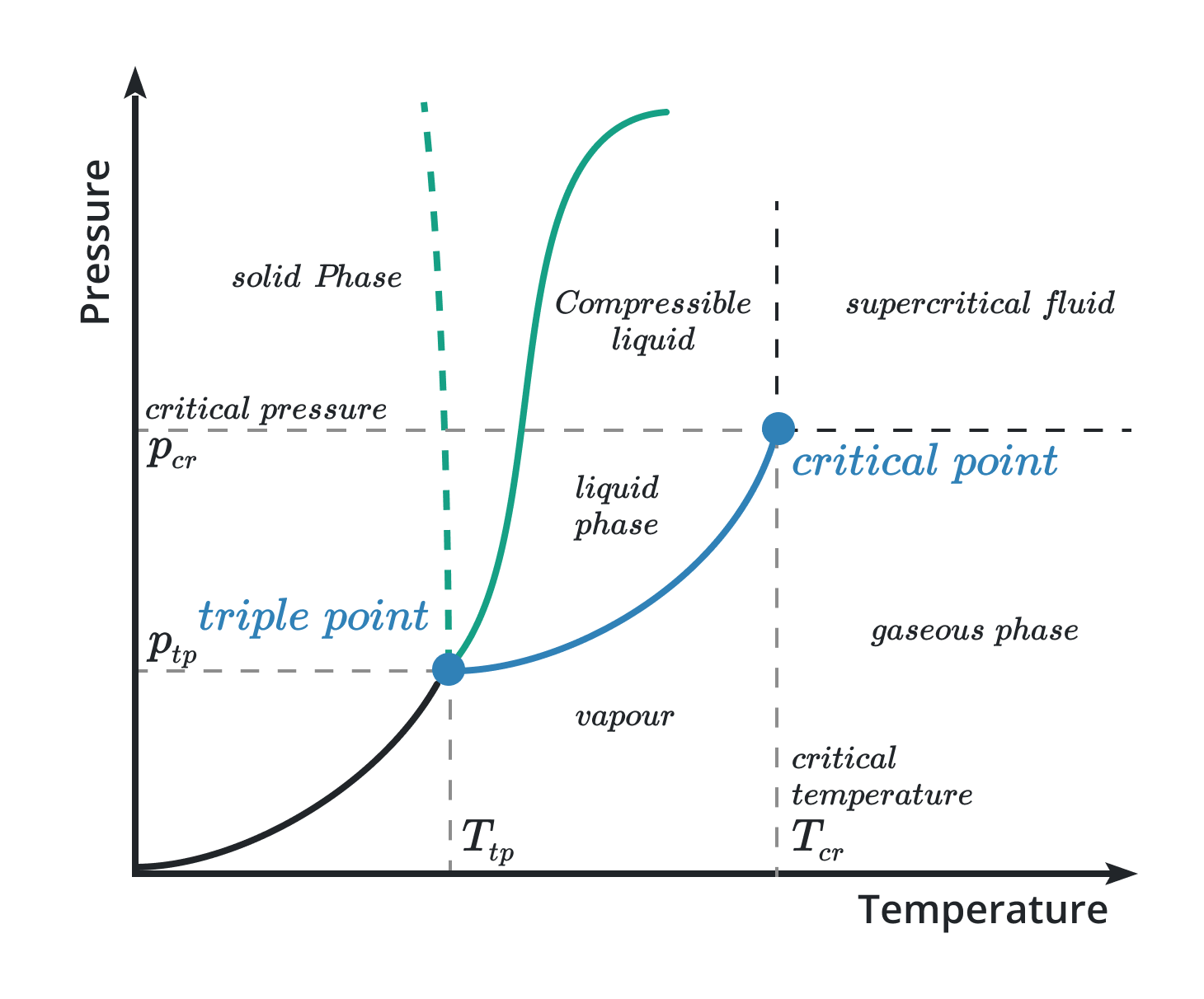# Critical Point Calculator

Enter the function and press calculate button to find the critical points

This will be calculated:

Give Us Feedback

## Critical points calculator with steps

Critical points calculator finds the values of single or multivariable functions. This critical number calculator determines those points on which the function is not differentiable.

## What is the critical point?

A critical point is the point of the function at which the differential of the function is zero or undefined. It can also be defined as a point on the graph of a function where the differentiation is zero or infinite.Critical points can be calculated by putting the first derivative equal to zero.

f'(x) = 0

## How to calculate the critical point?

To learn how to calculate the critical points, follow the below examples.

Example 1

Calculate the critical point of 3x^2 + 4x + 9.

Solution

Step I: First of all, find the first derivative of the given function.

d/dx [3x^2 + 4x + 9] = d/dx [3x^2] + d/dx [4x] + d/dx 

d/dx [3x^2 + 4x + 9] = 6x + 4 + 0

d/dx [3x^2 + 4x + 9] = 6x + 4

Step II: Now calculate the critical point by substituting the first derivative equal to zero.

d/dx [3x^2 + 4x + 9] = 0

6x + 4 = 0

6x = -4

x = -4/6 = -2/3

Hence, the critical point of the given function is x = -2/3

Example 2:

Calculate the critical point of 4x^2 + 6xy + 8y.

Solution

Step I: First of all, calculate the first partial derivative of the function w.r.t “x”.

∂/∂x [4x^2 + 6xy + 8y] = ∂/∂x [4x^2] + ∂/∂x [6xy] + ∂/∂x [8y]

∂/∂x [4x^2 + 6xy + 8y] = 8x + 6y + 0

∂/∂x [4x^2 + 6xy + 8y] = 8x + 6y

Step II: Now calculate the first partial derivative of the function w.r.t “y”.

∂/∂y [4x^2 + 6xy + 8y] = ∂/∂y [4x^2] + ∂/∂y [6xy] + ∂/∂y [8y]

∂/∂y [4x^2 + 6xy + 8y] = 0 + 6x + 8

∂/∂y [4x^2 + 6xy + 8y] = 6x + 8

Step III: Put the result of the first partial derivatives equal to zero.

For ∂/∂x [f(x, y)]

8x + 6y = 0

6y = -8x

y = -8x/6 … (i)

For ∂/∂y [f(x, y)]

6x + 8 = 0

6x = -8

x = -8/6 = -4/3

put the value of “x” in (i)

y = -8(-4/3)/6

y = 32/18

y = 16/9

Hence the critical points of the given function are:

x = -4/3

y = 16/9

### Math Tools